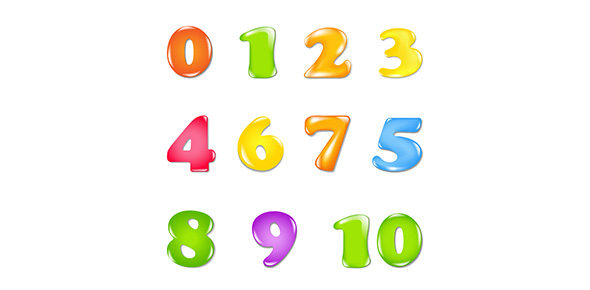# The Ultimate Quiz On Number

18 Questions | Total Attempts: 200SettingsIf you have the ability to understand the game of numbers then you should consider yourself one of the smartest people around as everything we do needs some calculations to understand. How well do you understand algebra and calculus and the different types of models in mathematics? If you believe you know much about these all you have to do is take the quiz below and prove it. Good luck!

• 1.
An expression that contains variables, numbers, and operations.
• A.

Math expression

• B.

Variable expression

• C.

Numerical expression

• 2.
A letter used to represent a number.
• A.

Exponent

• B.

Verbal model

• C.

Variable

• 3.
7 + 5 is an example of a:
• A.

Numerical expression

• B.

Variable expression

• C.

Verbal model

• 4.
When there is nothing between a number and a letter (like 4b) you should:
• A.

• B.

Subtract

• C.

Multiply

• D.

Divide

• 5.
To evaluate an expression means to:
• A.

Substitute a variable with a number and solve

• B.

Make sure that the expression is correct

• C.

None of the above

• 6.
How would you represent "Seven increased by a number"?
• A.

7 + n

• B.

7n

• C.

N7

• D.

7 - n

• 7.
How would you represent "The quotient of a number and 2"?
• A.

2n

• B.

N/2

• C.

(2 + n)

• D.

None of the above

• 8.
How would you represent "12 less than a number"
• A.

12 - n

• B.

12n

• C.

N - 12

• D.

N/12

• 9.
How would you represent "The product of 3 and a number plus 5"?
• A.

3(5) + n

• B.

N + 3 (5)

• C.

3n + 5

• D.

3xn + 5

• 10.
What is the difference between an expression and an equation?
• A.

An expression has an equal sign

• B.

An equation has an equal sign

• C.

There is no difference

• 11.
Evaluate the expression when x = 412x
• A.

16

• B.

3

• C.

48

• D.

8

• 12.
Evaluate the expression when x = 321/x
• A.

18

• B.

24

• C.

63

• D.

7

• 13.
Which expression would represent how much money you would make for a given amount of hours (h)  if you earned \$7.50 per hour?
• A.

\$7.50h

• B.

\$7.50 + h

• C.

\$7.50 / h

• 14.
Evaluate the expression when x = 5 and y= 4xy + x
• A.

20

• B.

24

• C.

25

• D.

30

• 15.
Evaluate the expression when x = 257 and y = 400y - x
• A.

43

• B.

143

• C.

243

• D.

257

• 16.
What is 2 to the 3rd power?
• A.

2

• B.

6

• C.

8

• D.

10

• 17.
An exponent tells how many times the base is:
• A.

• B.

Subtracted

• C.

Multiplied

• D.

Divided

• 18.
What is the only type of extra credit you can do for Mrs. Scarborough's class?
• A.

Give her compliments daily

• B.

Bring her chocolate

• C.

Redo tests and quizzes

Related TopicsBack to top